Dynamic format strings have been available with calculation groups for a long time. Recently, Power BI added the support of dynamic format strings to regular measures. At the time of writing, the feature is in preview and must be enabled in the Power BI options.

Once the feature is enabled, you need to use the Dynamic Format for a measure to enable the dynamic format string for the measure.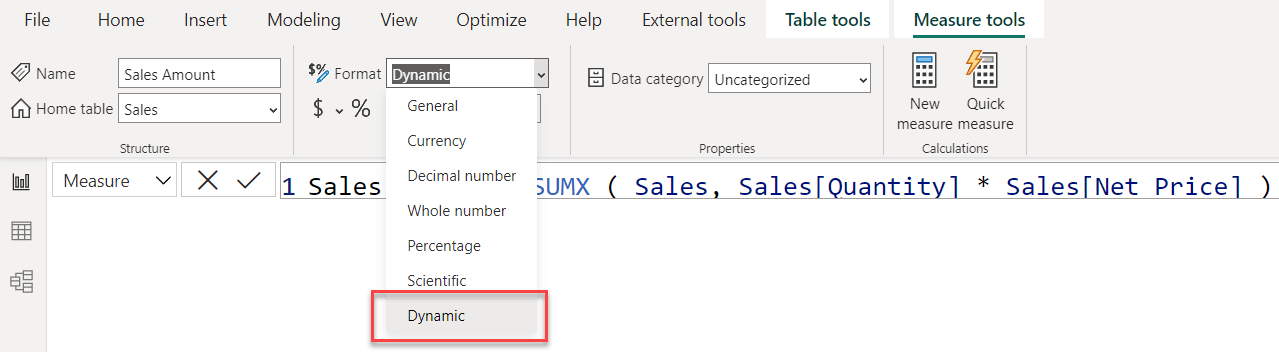Once the measure is in Dynamic format, you can choose “Format” from the dropdown menu on the left of the DAX code.Once Format is selected, you can write any DAX expression that will be evaluated as a format string. The DAX expression is not preceded by any assignment operator, as is the case when you define the measure.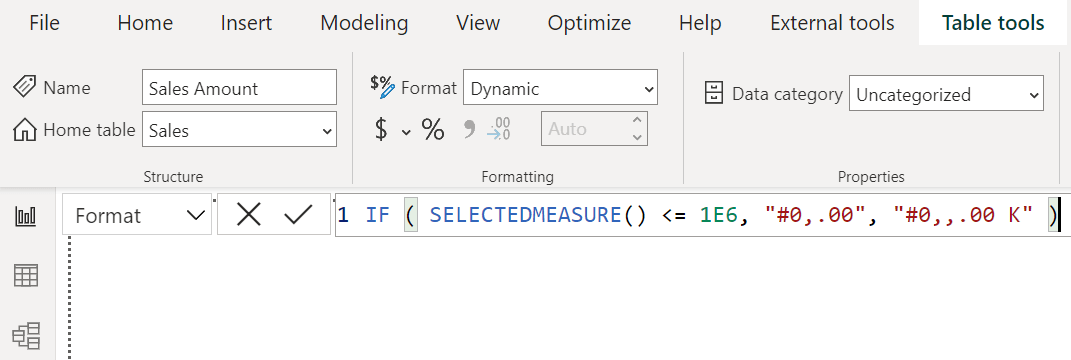The DAX expression needs to return a valid format string. The main advantage is that – being dynamic – it can change its value depending on the filter context or the value of the computed measure.

There are an incredible number of use cases for the feature. Here, we explore a couple of scenarios and dive into the implementation details.

The first example is to add the currency symbol to the sales amount. In the Sales table, a column contains the currency code of the transaction; the requirement is to build a report like the following.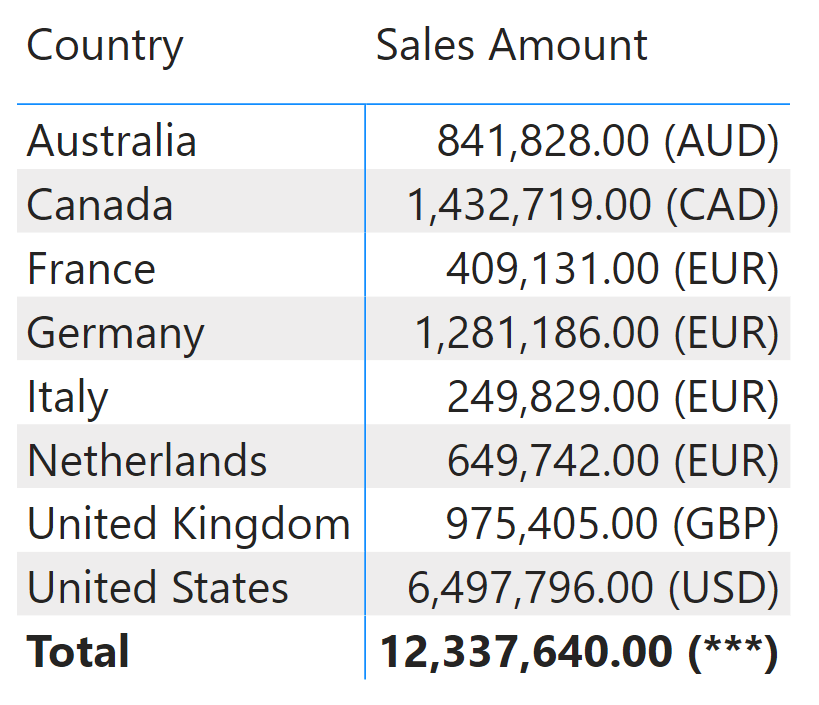In the format string code, we first retrieve the current value of the currency code and then build a suitable format string:

Format String Expression for Sales Amount measure in Sales table
```VAR BaseFormatString = "#0,0.00"
VAR CurrSymbol = SELECTEDVALUE ( Sales[Currency Code], "***")
VAR FormatString = BaseFormatString & " (" & CurrSymbol & ")"
RETURN
FormatString
```

Another helpful example of a format string is to reduce the number of digits shown, using K or M as units of measure for large values. This goal requires that you inspect the current value of the measure. If you need the current value of a measure – from inside a format string – you can use the SELECTEDMEASURE function. The following format string is an example of this:

Format String Expression for Sales Amount measure in Sales table
```VAR CurrentValue = SELECTEDMEASURE()
RETURN
SWITCH (
TRUE (),
// Special cases to force K and M in the sample report
CurrentValue <= 1E3, "#,0.00 K",
CurrentValue <= 1E6, "#,0,.00 K",
CurrentValue <= 1E9, "#,0,,.00 M",
CurrentValue <= 1E12, "#,0,,,.00 G"
)
```

The format string produces smaller numbers, with the correct unit of measure on each row.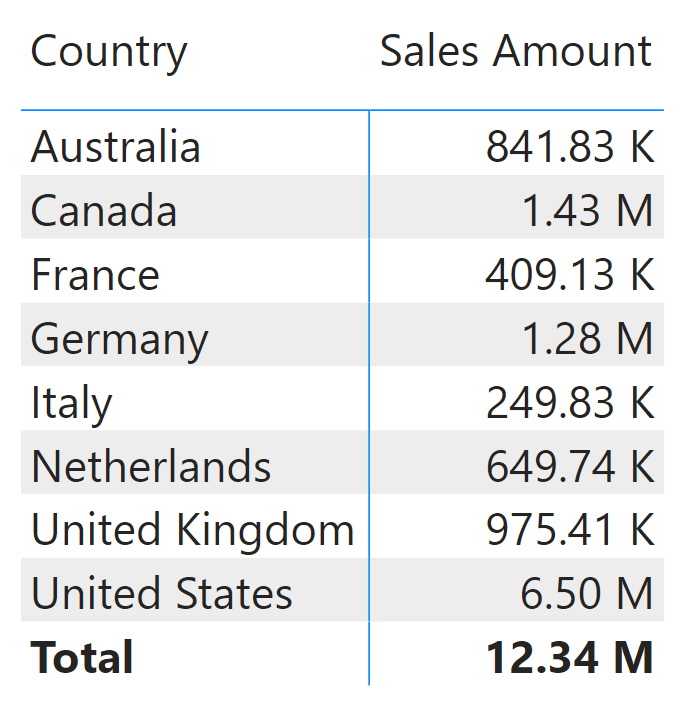However, there could be cases where the rows have the same symbol, and the total is different. We forced the result for this example.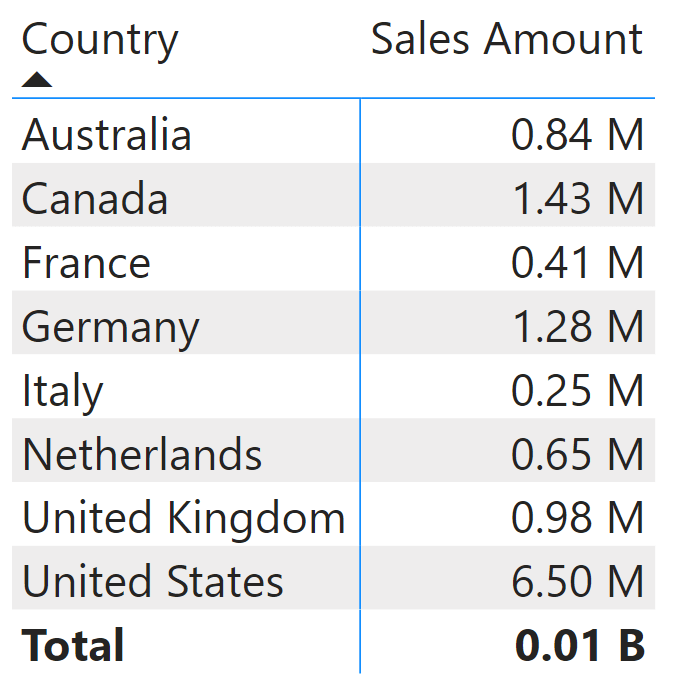Because the format string is chosen based on the individual cell values, different cells can use different units of measure. In our example, the total level displays billion (B) as the unit of measure, whereas individual rows use millions (M). If you want to avoid different units of measure being displayed in the same visual, you can base the unit of measure on the total and then use that unit of measure for all the rows:

Format String Expression for Sales Amount measure in Sales table
```VAR CurrentValue =
CALCULATE ( SELECTEDMEASURE (), ALLSELECTED () )
RETURN
SWITCH (
TRUE (),
CurrentValue <= 1E3, "#,0.00 K",
CurrentValue <= 1E6, "#,0,.00 K",
CurrentValue <= 1E9, "#,0,,.00 M",
CurrentValue <= 1E12, "#,0,,,.00 G"
)
```

Using this format string, all the rows use the same unit of measure, including the total.When the filter changes, the unit of measure changes for all the cells, including the total.## Optimizations

Now that we have a good idea about the feature let us dive into more technical details.

When a measure has a dynamic format string, the format string can be different for each row of the resultset. To obtain the correct format string for every row, Tabular uses an internal measure for every measure with a dynamic format string.

You can verify this behavior by using the performance analyzer to obtain the query executed to fill one of the previous matrixes:

```EVALUATE
SUMMARIZECOLUMNS (
"Sales_Amount", 'Sales'[Sales Amount],
"v_Sales_Amount_FormatString", IGNORE ( 'Sales'[_Sales Amount FormatString] )
)
```

The result contains both the value of the measure and its format string.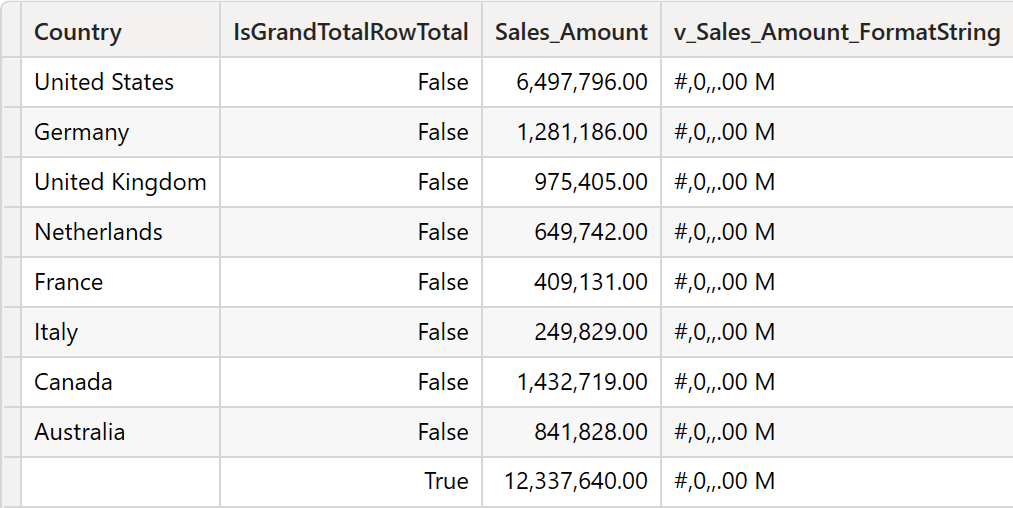The _Sales Amount FormatString measure is the internal measure that computes the format string for Sales Amount. The name for the internal measure is derived from the original name by prefixing an underscore (_) and adding the word FormatString at the end, after a space.

This naming pattern is reserved. If you try to create a measure with the same pattern, Power BI throws an error indicating that you cannot use that name. The internal measure exists only for those with a dynamic format string. Measures with static format strings do not create additional columns to the resultsets retrieved by Power BI.

The format string expression is stored as part of the model in the FormatStringExpression TOM property of a measure. This detail is crucial because a Power BI report using a live connection to a remote dataset cannot use dynamic format strings. Indeed, report measures created in live-connected models are executed as query measures, that is, measures defined in the query. Query measures do not have all the TOM properties; they only define the DAX code.

The fact that the internal measure handles a format string comes in handy when you need to debug the format string value or any performance issue in the format string. Indeed, every measure with a dynamic format string increases the complexity of the DAX query executed by Power BI. In general, format strings should be simple DAX expressions to get a fast execution. By authoring poor DAX code, you can make the performance of a query worse, just because of the dynamic format string.

Let us elaborate on this with an example. In one of the previous format strings, we used SELECTEDMEASURE to change the format string based on the value of the measure. An interesting question is: is the measure actually evaluated twice? Once for the value and once for the format string? With DAX Studio, the answer is quite simple to obtain. We only need to inspect the query plan of a simple query and check whether the code that computes the measure is duplicated.

To simplify the query plans, let us use a straightforward dynamic format string:

Format String Expression for Sales Amount measure in Sales table
```IF ( SELECTEDMEASURE() <= 1E6, "#0,.00", "#0,,.00 M" )
```

And then we execute this query:

```EVALUATE
SUMMARIZECOLUMNS (
"Sales_Amount", 'Sales'[Sales Amount],
"FormatString", 'Sales'[_Sales Amount FormatString]
)
```

The query plan shows that two datacaches are consumed by the formula engine.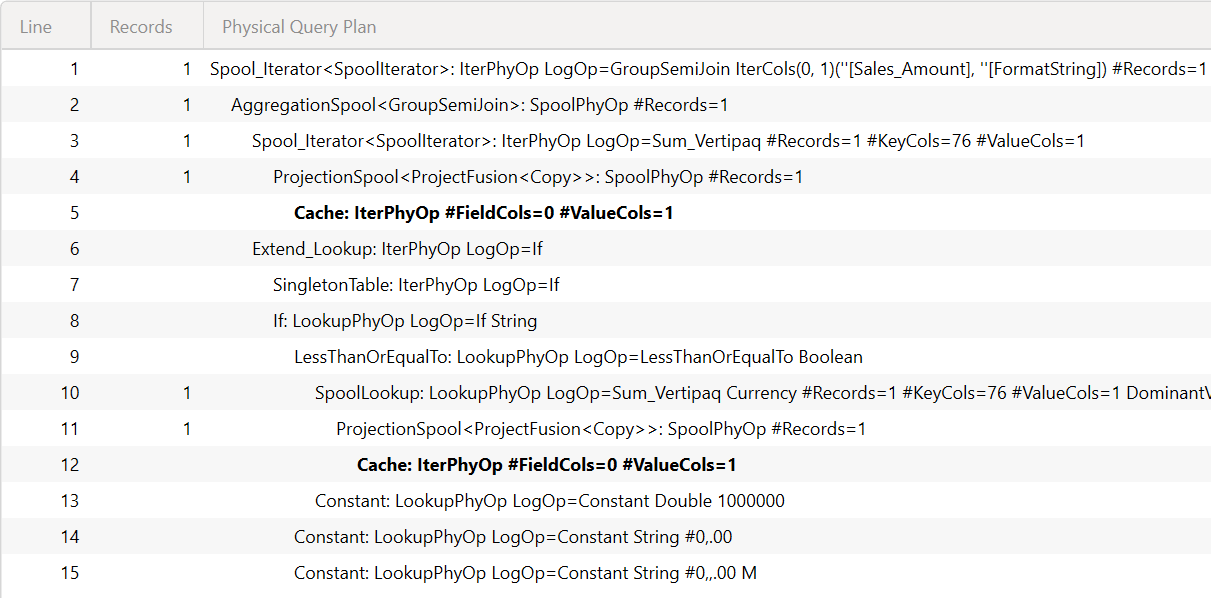However, the server timings panel shows a single storage engine query indicating that the two datacaches consume the result of a single storage engine query. Therefore, from the storage engine point of view, there is no additional cost by querying the SELECTEDMEASURE. What about the formula engine? The measure we are using right now retrieves values computed by the storage engine and uses them. However, a more complex (and realistic) measure would require some effort by the formula engine. Are the nodes of the formula engine duplicated or not? To test it, it is enough to add to the Sales Amount measure a piece of code that requires the intervention of the formula engine:

Measure in Sales table
```Sales Amount = TRUNC ( SUMX ( Sales, Sales[Quantity] * Sales[Net Price] ) )
```

The presence of TRUNC requires the intervention of the formula engine. Indeed, the storage engine computes the sales amount value, but the formula engine must execute the truncation. With this version of the measure, our previous query shows a different query plan.The two red boxes highlight the formula engine part of the measure: a datatype conversion and truncation.

Therefore, using SELECTEDMEASURE in the format string comes at a price. The formula engine part of the measure needs to be executed at least twice: once to compute the actual value and once to define the format string. For a formula engine-bound measure, that price can be extremely high.

Be mindful that the feature is currently in preview. Therefore, the code might still not be entirely optimized. However, these are the current findings, and we strongly suggest developers pay special attention to performance and monitor the time required to compute dynamic format strings.

## Internals

The format string expression can be referenced in DAX by using the fully qualified internal name created automatically. For example, consider the [Sales Amount] measure. The internal measure for the format string is [_Sales Amount FormatString]. However, to reference such an expression in DAX, the code must be Sales[_Sales Amount FormatString]. The format string reference must include the table name, using the fully qualified name, which is a bad practice in user-defined code. However, we expect that code to be present only in DAX queries generated by Power BI.

If the format string expression is defined for a measure, it can be rewritten as a query measure in a DAX query. However, the query measure for a format string expression cannot use SELECTEDMEASURE. The Define Measure feature in DAX Studio (from version 3.0.8) generates the code for both the measure and the format string expression, replacing any SELECTEDMEASURE function with the corresponding measure reference:

```DEFINE
---- MODEL MEASURES BEGIN ----
MEASURE Sales[Sales Amount] =
SUMX ( Sales, Sales[Quantity] * Sales[Net Price] )
MEASURE Sales[_Sales Amount FormatString] =
IF (
/* SELECTEDMEASURE() --> */ Sales[Sales Amount] /* <-| */ <= 1E6,
"#0,.00",
"#0,,.00 M"F
)
---- MODEL MEASURES END ----

EVALUATE
SUMMARIZECOLUMNS (
"Sales_Amount", 'Sales'[Sales Amount],
"FormatString", 'Sales'[_Sales Amount FormatString]
)
```

You should never reference a format string expression from another measure or format string expression: the expression is executed, but the SELECTEDMEASURE function always invokes the original measure. For example, consider the following format strings for Sales Amount and Margin:

Format String Expression for Sales Amount measure in Sales table
```IF ( SELECTEDMEASURE() <= 1E6, "#0,.00", "#0,,.00 M" )
```
Format String Expression for Margin measure in Sales table
```Sales[_Sales Amount FormatString]
```

The SELECTEDMEASURE function in the Sales Amount format string always references the Sales Amount measure, also when it is invoked through the Margin format string. As a result, the Margin for Canada and Germany is formatted with M as Sales Amount, ignoring the smaller value returned by Margin that should have another format string.## Conclusions

Dynamic format strings are a welcome addition to the reporting features of Power BI and Tabular. As always, adding features comes at a cost. There are no issues for simple dynamic format strings: the time required to compute the format strings is tiny and can be safely ignored. However, if the format string invokes other measures or uses SELECTEDMEASURE to invoke the current measure, you might face performance issues.

As always, the task of a BI developer is to find the correct balance between usability and performance. Dynamic format strings add another variable to the complex equation we need to solve every day. At the same time, they provide tremendous power for many reports.

SELECTEDMEASUREReturns the measure that is currently being evaluated.

`SELECTEDMEASURE ( )`

TRUNCTruncates a number to an integer by removing the decimal, or fractional, part of the number.

`TRUNC ( <Number> [, <NumberOfDigits>] )`